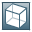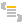Show TOC

###enum RFCTYPEDefines RFC parameter types for each corresponding ABAP types.

#### StructureSyntax

1. `typedef enum _RFCTYPE`
2. `{`
3. `  RFCTYPE_CHAR   = 0,		/* 1-byte or multibyte character, fixed sized, blank padded */`
4. `  RFCTYPE_DATE   = 1,		/* date ( YYYYYMMDD ) */`
5. `  RFCTYPE_BCD    = 2,		/* packed number, any length between 1 and 16 bytes */`
6. `  RFCTYPE_TIME   = 3,		/* time (HHMMSS)  */`
7. `  RFCTYPE_BYTE   = 4,		/* raw data, binary, fixed length, zero padded.*/`
8. `  RFCTYPE_TABLE   = 5,		/* internal table */`
9. `  RFCTYPE_NUM    = 6,		/* digits, fixed size, leading '0' padded. */`
10. `  RFCTYPE_FLOAT  = 7,		/* floating point, double precission */`
11. `  RFCTYPE_INT    = 8,		/* 4-byte integer */`
12. `  RFCTYPE_INT2   = 9,		/* 2-byte integer, obsolete, not directly supported by ABAP/4 */`
13. `  RFCTYPE_INT1   = 10,		/* 1-byte integer, obsolete, not directly supported by ABAP/4 */`
14. `  RFCTYPE_NULL  = 14,		/* not supported data type. */`
15. `  RFCTYPE_STRUCTURE = 17,	/* abap structure */`
16. `  RFCTYPE_DECF16  = 23,      /* 16-bytes decimal float point */`
17. `  RFCTYPE_DECF32  = 24,      /* 32-bytes decimal float point */`
18. `  RFCTYPE_XMLDATA = 28,      /* variable-length, zero terminated string representing ABAP data   with hierarchical structure (so-called TYPE2) as XML */`
19. `  RFCTYPE_STRING = 29,      /* variable-length, zero terminated string */`
20. `  RFCTYPE_XSTRING = 30,     /* variable-length raw string, length in bytes */`
21. `  RFCTYPE_EXCEPTION = 98,`
22. `  _RFCTYPE_max_value      /* the max. value of RFCTYPEs */`
23. `}RFCTYPE;`
End of the code.